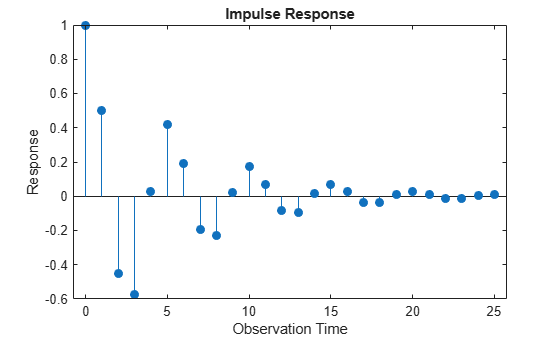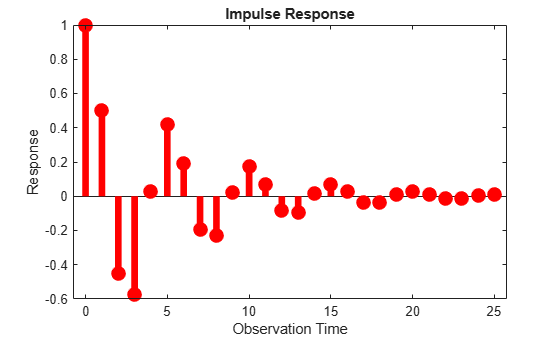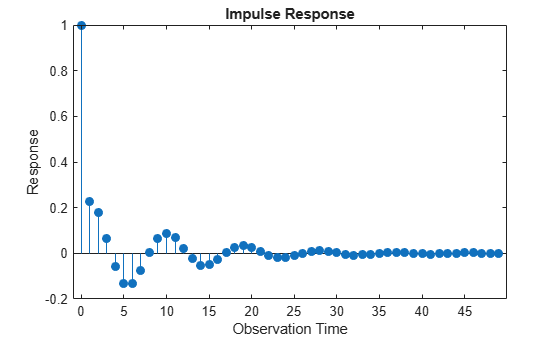# impulse

Generate univariate ARIMA model impulse response function (IRF)

## Syntax

``impulse(Mdl)``
``impulse(Mdl,numObs)``
``y = impulse(___)``

## Description

`impulse` generates, or plots, the impulse response function (IRF) of a univariate autoregressive integrated moving average (ARIMA) process specified by an `arima` model object.

Alternatively, you can use `armairf` to generate or plot the IRF of an ARMA process specified by AR and MA lag operator polynomial coefficients.

example

``impulse(Mdl)` plots a discrete stem plot of the IRF of the univariate ARIMA model `Mdl` to the current figure window. `impulse` plots the dynamic responses starting at period 0, during which `impulse` applies a unit shock to the innovation.`

example

``impulse(Mdl,numObs)` plots the `numObs` dynamic responses from periods 0 through `numObs` – 1.`

example

``y = impulse(___)` returns the IRF `y` of the ARIMA model, using any of the input argument combinations in the previous syntaxes.`

## Examples

collapse all

Create the AR(2) model

`${y}_{t}=0.5{y}_{t-1}-0.7{y}_{t-2}+{\epsilon }_{t},$`

where ${\epsilon }_{\mathit{t}}$ is a standard Gaussian process.

`Mdl = arima('AR',{0.5,-0.7},'Constant',0)`
```Mdl = arima with properties: Description: "ARIMA(2,0,0) Model (Gaussian Distribution)" SeriesName: "Y" Distribution: Name = "Gaussian" P: 2 D: 0 Q: 0 Constant: 0 AR: {0.5 -0.7} at lags [1 2] SAR: {} MA: {} SMA: {} Seasonality: 0 Beta: [1×0] Variance: NaN ```

Plot the IRF of ${\mathit{y}}_{\mathit{t}}$.

`impulse(Mdl)`The IRF has length 26; it begins at period 0, during which `impulse` applies a unit shock to the innovation, and ends at period 25. `impulse` computes the IRF by inverting the underlying AR lag operator polynomial. The length of the IRF is 26 because the dynamic multipliers beyond period 25 are below the division algorithm tolerances.

The model is stationary; the impulse response function decays with a sinusoidal pattern.

You can change characteristics of the plot by adjusting the properties of the underlying stem plot. The axes handle object stores the stem plot handle in the `Children` property.

Increase the line thickness (the default is 0.5). Also, change the color of the stem plot to red by using the RGB color value.

```h = gca; % Current axes handle stemh = h.Children; stemh.LineWidth = 5; stemh.Color = [1 0 0];```Load the quarterly US GDP data set.

`load Data_GDP`

For details on the data, enter `Description` at the command line.

Compute the GDP growth rate.

`y = price2ret(Data);`

Consider an ARMA(2,2) model for the GDP rate series. Create an `arima` model template for estimation.

`Mdl = arima(2,0,2)`
```Mdl = arima with properties: Description: "ARIMA(2,0,2) Model (Gaussian Distribution)" SeriesName: "Y" Distribution: Name = "Gaussian" P: 2 D: 0 Q: 2 Constant: NaN AR: {NaN NaN} at lags [1 2] SAR: {} MA: {NaN NaN} at lags [1 2] SMA: {} Seasonality: 0 Beta: [1×0] Variance: NaN ```

`NaN` values in properties are placeholders for estimable model parameters.

Fit the model to the entire series.

`EstMdl = estimate(Mdl,y)`
``` ARIMA(2,0,2) Model (Gaussian Distribution): Value StandardError TStatistic PValue __________ _____________ __________ __________ Constant 0.0036702 0.00058179 6.3084 2.8189e-10 AR{1} 1.372 0.089006 15.415 1.3064e-53 AR{2} -0.8069 0.069497 -11.611 3.6432e-31 MA{1} -1.1431 0.10159 -11.253 2.2483e-29 MA{2} 0.67355 0.08512 7.9129 2.5155e-15 Variance 8.3071e-05 5.9331e-06 14.001 1.5322e-44 ```
```EstMdl = arima with properties: Description: "ARIMA(2,0,2) Model (Gaussian Distribution)" SeriesName: "Y" Distribution: Name = "Gaussian" P: 2 D: 0 Q: 2 Constant: 0.00367019 AR: {1.37199 -0.806899} at lags [1 2] SAR: {} MA: {-1.14315 0.673546} at lags [1 2] SMA: {} Seasonality: 0 Beta: [1×0] Variance: 8.30707e-05 ```

The estimated AR(2) model of the GDP rate series is

`${y}_{t}=0.004+1.372{y}_{t-1}-0.807{y}_{t-2}+{\epsilon }_{t}-1.143{\epsilon }_{t-1}+0.674{\epsilon }_{t-2},$`

where ${\epsilon }_{\mathit{t}}$ is a Gaussian series with mean 0 and variance 0.00008.

Compute the IRF of the estimated model for 50 periods.

`impulse(EstMdl,50)`The dynamic response to the initial innovation shock dissipates after about 35 quarters.

Create the ARMA(1,1) model

`${y}_{t}=0.7{y}_{t-1}+{\epsilon }_{t}+0.2{\epsilon }_{t-1}.$`

`Mdl = arima('AR',0.7,'MA',0.2,'Constant',0);`

Return the IRF for 15 periods.

```numObs = 15; periods = 0:(numObs-1); y = impulse(Mdl,numObs); irf = table(periods',y,'VariableNames',["Period" "y"])```
```irf=15×2 table Period y ______ ________ 0 1 1 0.9 2 0.63 3 0.441 4 0.3087 5 0.21609 6 0.15126 7 0.10588 8 0.074119 9 0.051883 10 0.036318 11 0.025423 12 0.017796 13 0.012457 14 0.00872 ```

`y(0)`, which is the dynamic response of the system at the time `impulse` shocks the innovation, is `1`.

## Input Arguments

collapse all

Fully specified ARIMA model, specified as an `arima` model object created by `arima` or `estimate`.

The properties of `Mdl` cannot contain `NaN` values.

Number of periods to include in the IRF (the number of periods for which `impulse` computes the IRF), specified as a positive integer.

If you specify `numObs`, `impulse` computes the IRF by filtering a unit impulse, followed by a vector of zeros of length `numObs` – 1, through the model `Mdl`. In this case, the filtering algorithm is efficient.

By default, `impulse` determines the length of the IRF by implementing the following algorithm:

1. Represent the ARIMA process as a pure MA process by applying lag operator polynomial division.

2. Truncate the resulting MA polynomial by enforcing the default tolerances.

For more details, see `mldivide`.

Tip

• If `Mdl` contains an AR or differencing polynomial (seasonal or nonseasonal) the MA representation of the model has infinite lag order. Consider specifying `numObs` in this case.

• If `Mdl` contains only MA polynomials, the IRF has length `Mdl.Q + 1`.

Example: `10`

Data Types: `double`

## Output Arguments

collapse all

IRF, returned as a numeric column vector. If you specify `numObs`, `Y` has length `numObs`. If you do not specify `numObs`, the tolerances of the underlying lag operator polynomial division algorithm determine the length of `y`.

`y(j)` is the impulse response of yt at period `j` – 1. `y(0)` represents the impulse response during the period in which `impulse` applies the unit shock to the innovation (ε0 = 1).

collapse all

### Impulse Response Function

The impulse response function (IRF) of a univariate ARIMAX process is the dynamic response of the system to a single impulse (innovation shock).

Consider an ARIMAX model expressed as an MA process

`${y}_{t}={m}_{t}+\psi \left(L\right){\epsilon }_{t},$`

where:

• ψ(L) is the infinite-degree MA lag operator polynomial ${\psi }_{0}+{\psi }_{1}L+{\psi }_{2}{L}^{2}+\dots$ with scalar coefficients ψj, j = 0,1,2,… and ψ0 = 1.

• mt is the deterministic, innovation-free conditional mean of the process at time t.

The IRF measures the change to the response j periods in the future due to a change in the innovation at time t, for j = 0,1,2,…. Symbolically, the IRF at period j is

`$\frac{\partial {y}_{t+j}}{\partial {\epsilon }_{t}}={\psi }_{j}.$`

The sequence of dynamic multipliers , ψ0, ψ1, ψ2,..., measures the sensitivity of the process to a purely transitory change in the innovation process, with past responses and future innovations set to 0. Because the partial derivative is taken with respect to the innovation, the presence of deterministic terms in the model, such as the constant and the exogenous regression component, has no effect on the impulse responses.

Properties of the IRF determine characteristics of the process:

• If the sequence $\left\{{\psi }_{j}\right\}$ is absolutely summable, yt is a covariance-stationary stochastic process . For a stationary stochastic process, the impact on the process due to a change in εt is not permanent, and the effect of the impulse decays to zero.

• Otherwise, the process yt is nonstationary, and a change in εt affects the process permanently.

Because innovations can be interpreted as one-step-ahead forecast errors, the impulse response is also known as the forecast error impulse response.

## Tips

• To improve performance of the filtering algorithm, specify the number of periods to include in the IRF `numObs`. When you do not specify `numObs`, `impulse` computes the IRF by using the lag operator polynomial division algorithm, which is relatively slow, to represent the input model `Mdl` as a truncated, infinite-degree, moving average model. The length of the resulting IRF is generally unknown.

 Box, George E. P., Gwilym M. Jenkins, and Gregory C. Reinsel. Time Series Analysis: Forecasting and Control. 3rd ed. Englewood Cliffs, NJ: Prentice Hall, 1994.

 Enders, Walter. Applied Econometric Time Series. Hoboken, NJ: John Wiley & Sons, Inc., 1995.

 Hamilton, James D. Time Series Analysis. Princeton, NJ: Princeton University Press, 1994.

 Lütkepohl, Helmut. New Introduction to Multiple Time Series Analysis. New York, NY: Springer-Verlag, 2007.

 Wold, Herman. "A Study in the Analysis of Stationary Time Series." Journal of the Institute of Actuaries 70 (March 1939): 113–115. https://doi.org/10.1017/S0020268100011574.